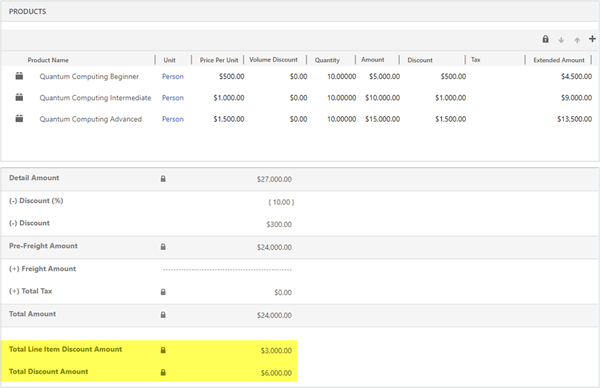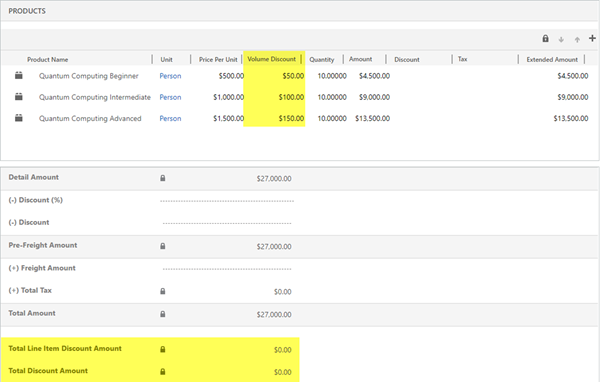# System Calculated Fields - Dynamics 365 Quote and Order Discounts

### Colin Maitland, 20 August 2018

In the third part of this three-part series I will describe two system calculated fields for Quotes/Orders that provide system calculated totals for the discounts that have been applied to the Quote/Order.

These two fields are not displayed on the Order form but can be added to the Order form if required. They are:

• •    Total Line Item Discount Amount: is the system calculated total Manual Discount Amount from the Quote/Order Products.
• •    Total Discount Amount: is the Total Line Item Discount Amount + Discount Percent (calculated discount amount) + Discount Amount from the Order.These totals do not include the any discounts applied to an Order using Volume Discounts.